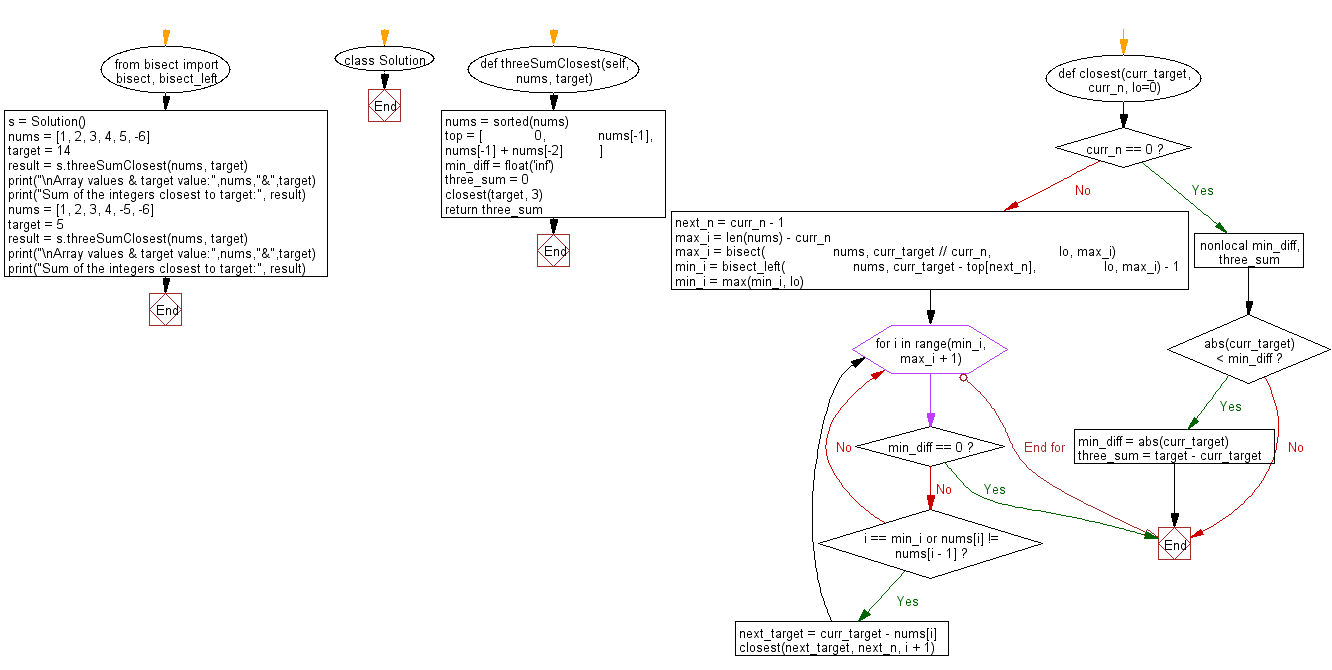﻿ Python Bisect: Find a triplet in an array such that the sum is closest to a given number - w3resource# Python Bisect: Find a triplet in an array such that the sum is closest to a given number

## Python Bisect: Exercise-8 with Solution

Write a Python program to find a triplet in an array such that the sum is closest to a given number. Return the sum of the three integers.

Sample Solution:

Python Code:

``````#Source: https://bit.ly/2SRefdb
from bisect import bisect, bisect_left
class Solution:
def threeSumClosest(self, nums, target):
"""
:type nums: List[int]
:type target: int
:rtype: int
"""
nums = sorted(nums)
# Let top[i] be the sum of largest i numbers.
top = [
0,
nums[-1],
nums[-1] + nums[-2]
]
min_diff = float('inf')
three_sum = 0
# Find range of the least number in curr_n (0, 1, 2 or 3)
# numbers that sum up to curr_target, then find range of
# 2nd least number and so on by recursion.
def closest(curr_target, curr_n, lo=0):
if curr_n == 0:
nonlocal min_diff, three_sum
if abs(curr_target) < min_diff:
min_diff = abs(curr_target)
three_sum = target - curr_target
return

next_n = curr_n - 1
max_i = len(nums) - curr_n
max_i = bisect(
nums, curr_target // curr_n,
lo, max_i)
min_i = bisect_left(
nums, curr_target - top[next_n],
lo, max_i) - 1
min_i = max(min_i, lo)

for i in range(min_i, max_i + 1):
if min_diff == 0:
return
if i == min_i or nums[i] != nums[i - 1]:
next_target = curr_target - nums[i]
closest(next_target, next_n, i + 1)

closest(target, 3)
return three_sum

s = Solution()
nums = [1, 2, 3, 4, 5, -6]
target = 14
result = s.threeSumClosest(nums, target)
print("\nArray values & target value:",nums,"&",target)
print("Sum of the integers closest to target:", result)

nums = [1, 2, 3, 4, -5, -6]
target = 5
result = s.threeSumClosest(nums, target)
print("\nArray values & target value:",nums,"&",target)
print("Sum of the integers closest to target:", result)
```
```

Sample Output:

```Array values & target value: [1, 2, 3, 4, 5, -6] & 14
Sum of the integers closest to target: 12

Array values & target value: [1, 2, 3, 4, -5, -6] & 5
Sum of the integers closest to target: 6
```

Flowchart:## Visualize Python code execution:

The following tool visualize what the computer is doing step-by-step as it executes the said program:

Python Code Editor:

Next: Write a Python program to find four elements from a given array of integers whose sum is equal to a given number. The solution set must not contain duplicate quadruplets.

What is the difficulty level of this exercise?

Test your Programming skills with w3resource's quiz.

﻿

## Python: Tips of the Day

How to make a chain of function decorators?

```from functools import wraps

def makebold(fn):
@wraps(fn)
def wrapped(*args, **kwargs):
return "<b>" + fn(*args, **kwargs) + "</b>"
return wrapped

def makeitalic(fn):
@wraps(fn)
def wrapped(*args, **kwargs):
return "<i>" + fn(*args, **kwargs) + "</i>"
return wrapped

@makebold
@makeitalic
def hello():
return "hello world"

@makebold
@makeitalic
def log(s):
return s

print hello()        # returns "<b><i>hello world</i></b>"
print hello.__name__ # with functools.wraps() this returns "hello"
print log('hello')   # returns "<b><i>hello</i></b>"
```

Ref: https://bit.ly/3cVz5iw